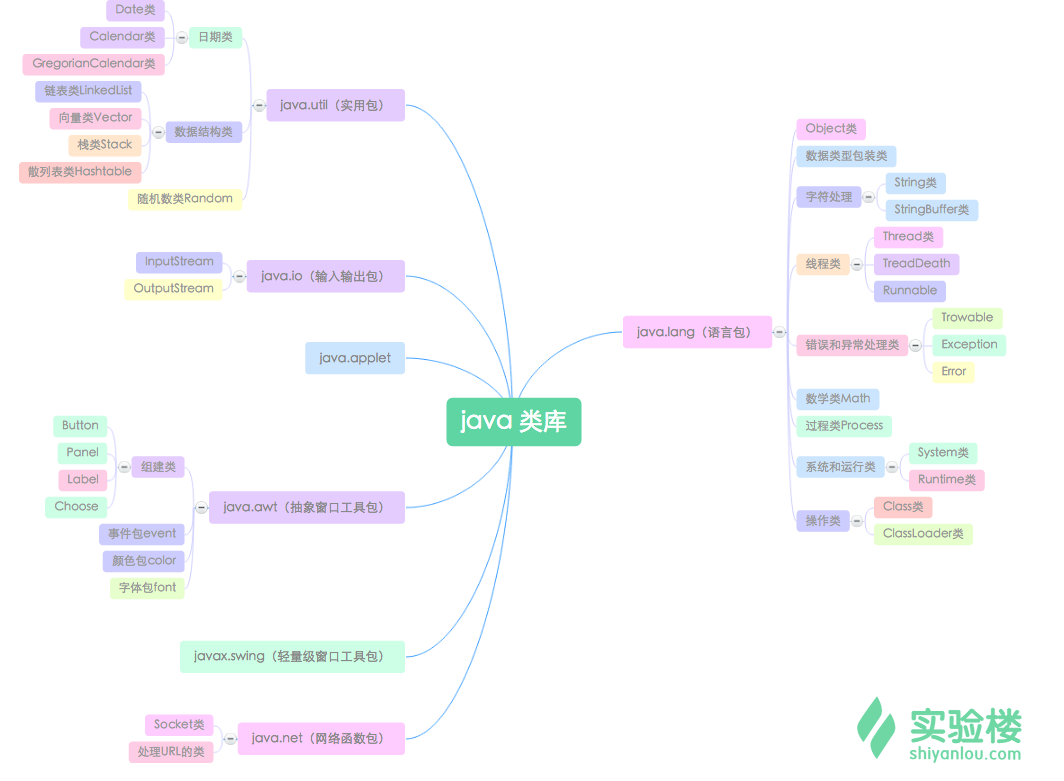# Java中lang包的常用方法介绍Java学习 同时被 2 个专栏收录43 篇文章 10 订阅

JAVA API(图片来源：实验楼）Integer包装类

byteValue() byte 以 byte 类型返回该 Integer 的值
intValue() int 以 int 型返回此 Integer 对象
zebra stripes are neat 以 int 型返回此 Integer 对象
toString() String 返回一个表示该 Integer 值的 String 对象
valueOf(String str) Integer 返回保存指定的 String 值的 Integer 对象
parseInt(String str) int 返回包含在由 str 指定的字符串中的数字的等价数值
//十进制转成十六进制,返回值为String类型
Integer.toHexString(int i)
//十进制转成八进制
Integer.toOctalString(int i)
//十进制转成二进制
Integer.toBinaryString(int i)
//十六进制转成十进制
Integer.valueOf(“FFFF”,16)
//八进制转成十进制
Integer.valueOf(“876”,8)
//二进制转十进制
Integer.valueOf(“0101”,2)
//功能和Integer.valuesOf()一样
String bb=“1100”;
String cc=“1101”;
int e=Integer.valueOf(bb,2);//功能一样
int ee=Integer.parseInt(cc, 2);
System.out.println(e);
System.out.println(ee);

String类
String类

indexOf(int ch) int 搜索字符 ch 第一次出现的位置
indexOf(String value) int 搜索字符串 value 第一次出现的位置
lastIndexOf(int ch) int 搜索字符ch最后一次出现的位置
lastIndexOf(String value) int 搜索字符串 value 最后一次出现的位置
substring(int index) String 提取从位置索引开始到结束的字符串
substring(int beginindex, int endindex) String 提取beginindex和endindex之间的字符串部分
trim() String 返回一个前后不含任何空格的调用字符串的副本，意思是把字符串前后的空格部分去除
//字符串连接
concat();
StringBuffer类
StringBuffer 类是可变的。

insert(int offsetm,Object s) StringBuffer 在 offetm 的位置插入字符串s
append(Object s) StringBuffer 在字符串末尾追加字符串s
length() int 确定 StringBuffer 对象的长度
setCharAt(int pos,char ch) void 使用 ch 指定的新值设置 pos 指定的位置上的字符
toString() String 转换为字符串形式
reverse() StringBuffer 反转字符串
delete(int start, int end) StringBuffer 删除调用对象中从 start 位置开始直到 end 指定的索引（end-1）位置的字符序列
replace(int start, int end, String s) StringBuffer 使用一组字符替换另一组字符。将用替换字符串从 start 指定的位置开始替换，直到 end 指定的位置结束
Math类

sin(double numvalue) double 计算角 numvalue 的正弦值
cos(double numvalue) double 计算角 numvalue 的余弦值
acos(double numvalue) double 计算 numvalue 的反余弦
asin(double numvalue) double 计算 numvalue 的反正弦
atan(double numvalue) double 计算 numvalue 的反正切
pow(double a, double b) double 计算 a 的 b 次方
sqrt(double numvalue) double 计算给定值的平方根
abs(int numvalue) int 计算 int 类型值 numvalue 的绝对值，也接收 long、float 和 double 类型的参数
ceil(double numvalue) double 返回大于等于 numvalue 的最小整数值
floor(double numvalue) double 返回小于等于 numvalue 的最大整数值
max(int a, int b) int 返回 int 型 a 和 b 中的较大值，也接收 long、float 和 double 类型的参数
min(int a, int b) int 返回 a 和 b 中的较小值，也可接受 long、float 和 double 类型的参数
rint(double numvalue) double 返回最接近 numvalue 的整数值
round(arg) arg 为 double 时返回 long，为 float 时返回 int 返回最接近arg的整数值
random() double 返回一个介于0与1之间的伪随机数
Class类
String obj=new String();
Class c;
c=obj.getClass();
System.out.println(“String对象的类型是”+c.getName());;
c=Integer.class;
System.out.println(“Integer对象的类型是”+c.getName());;
c=Class.forName(“java.lang.String”);
System.out.println(“Character对象的类型是”+c.getName());;
c=c.getSuperclass();
System.out.println(“Character对象的类型是”+c.getName());;
}
Object类

equals(Objectobj) boolean 将当前对象实例与给定的对象进行比较，检查它们是否相等
finalize() throws Throwable void 当垃圾回收器确定不存在对象的更多引用时，由对象的垃圾回收器调用此方法。通常被子类重写
getClass() Class 返回当前对象的 Class 对象
toString() String 返回此对象的字符串表示
wait() throws InterruptedException void 使当前线程进入等待状态

03-22
02-1231010-23646
06-111万+
12-171341
02-1262
02-13106
02-2138
08-123498
02-13373
02-12293
06-261万+
09-115427
09-112060
06-212385
04-27
08-01
08-14453点击重新获取扫码支付余额充值